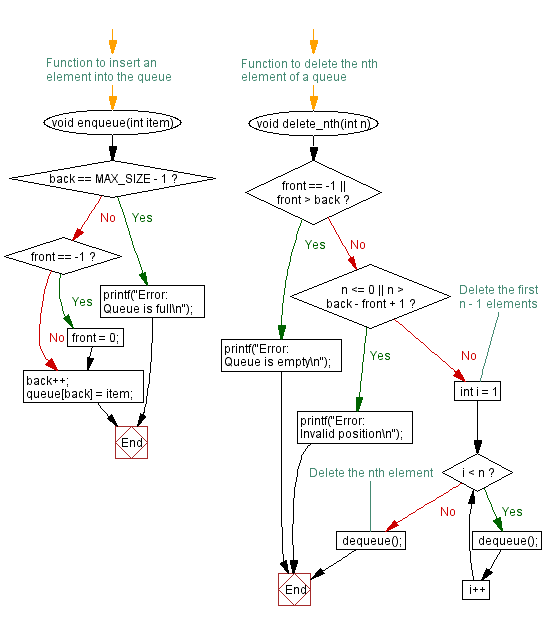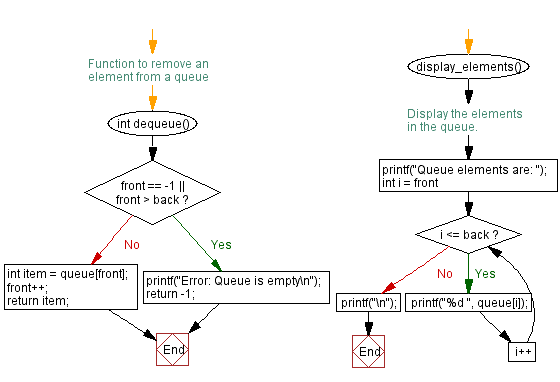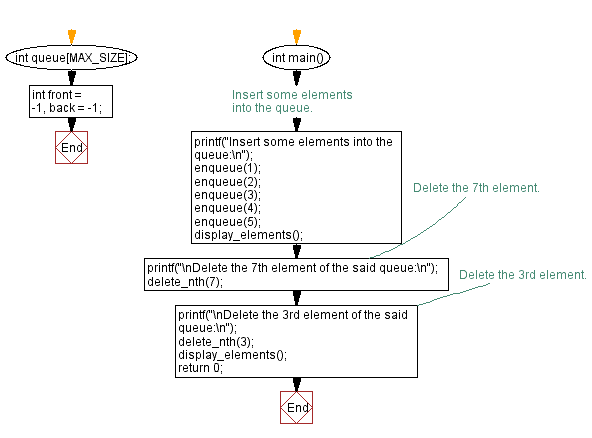﻿ C - Delete the nth element of a queue

# C Exercises: Delete the nth element of a queue

## C Queue: Exercise-11 with Solution

Write a C program to delete the nth element of a queue.

Firstly, this code checks if the queue is empty or if the position to delete is invalid. If the position is valid, it deletes the first n-1 elements, then deletes the nth element by calling the dequeue() function.

Sample Solution:

C Code:

``````#include <stdio.h>

#define MAX_SIZE 100

int queue[MAX_SIZE];
int front = -1, back = -1;

// Function to insert an element into the queue
void enqueue(int item) {
if (back == MAX_SIZE - 1) {
printf("Error: Queue is full\n");
return;
}
if (front == -1) {
front = 0;
}
back++;
queue[back] = item;
}

// Function to remove an element from a queue
int dequeue() {
if (front == -1 || front > back) {
printf("Error: Queue is empty\n");
return -1;
}
int item = queue[front];
front++;
return item;
}

// Function to delete the nth element of a queue
void delete_nth(int n) {
if (front == -1 || front > back) {
printf("Error: Queue is empty\n");
return;
}
if (n <= 0 || n > back - front + 1) {
printf("Error: Invalid position\n");
return;
}

// Delete the first n - 1 elements
for (int i = 1; i < n; i++) {
dequeue();
}

// Delete the nth element
dequeue();
}
display_elements()
{
// Display the elements in the queue.
printf("Queue elements are: ");
for (int i = front; i <= back; i++) {
printf("%d ", queue[i]);
}
printf("\n");
}

int main() {
// Insert some elements into the queue.
printf("Insert some elements into the queue:\n");
enqueue(1);
enqueue(2);
enqueue(3);
enqueue(4);
enqueue(5);
display_elements();
// Delete the 7th element.
printf("\nDelete the 7th element of the said queue:\n");
delete_nth(7);
// Delete the 3rd element.
printf("\nDelete the 3rd element of the said queue:\n");
delete_nth(3);
display_elements();

return 0;
}
```
```

Sample Output:

```Insert some elements into the queue:
Queue elements are: 1 2 3 4 5

Delete the 7th element of the said queue:
Error: Invalid position

Delete the 3rd element of the said queue:
Queue elements are: 4 5
```

Flowchart:C Programming Code Editor:

Have another way to solve this solution? Contribute your code (and comments) through Disqus.

What is the difficulty level of this exercise?

Test your Programming skills with w3resource's quiz.

﻿

## C Programming: Tips of the Day

C Programming - How do you pass a function as a parameter in C?

Declaration

A prototype for a function which takes a function parameter looks like the following:

```void func ( void (*f)(int) );
```

This states that the parameter f will be a pointer to a function which has a void return type and which takes a single int parameter. The following function (print) is an example of a function which could be passed to func as a parameter because it is the proper type:

```void print ( int x ) {
printf("%d\n", x);
}
```

Function Call

When calling a function with a function parameter, the value passed must be a pointer to a function. Use the function's name (without parentheses) for this:

```func(print);
```

would call func, passing the print function to it.

Function Body

As with any parameter, func can now use the parameter's name in the function body to access the value of the parameter. Let's say that func will apply the function it is passed to the numbers 0-4. Consider, first, what the loop would look like to call print directly:

```for ( int ctr = 0 ; ctr < 5 ; ctr++ ) {
print(ctr);
}
```

Since func's parameter declaration says that f is the name for a pointer to the desired function, we recall first that if f is a pointer then *f is the thing that f points to (i.e. the function print in this case). As a result, just replace every occurrence of print in the loop above with *f:

```void func ( void (*f)(int) ) {
for ( int ctr = 0 ; ctr < 5 ; ctr++ ) {
(*f)(ctr);
}
}
```

Ref : https://bit.ly/3skw9Um